# Chambers

## Chamber General Description and Quick Guide

In Flow Simulator, chambers represent regions of essentially constant fluid properties including flow velocity and vector direction. They serve as connection points for the Flow Simulator elements. Each chamber collects flow from all elements entering that chamber and discharges flow to all elements leaving that chamber. Nominally the elements having the chamber designated as the "downstream chamber" supply the entering flow and the elements having the chamber designated as the "upstream chamber" receive the flow leaving the chamber. However, it is possible that these roles will change as the direction of flow in some elements may reverse when Flow Simulator balances out the chamber pressures during the iterations. Flow Simulator permits no mass flow accumulation in chambers; use accumulators and tanks instead of chambers when transient behavior is required. Hence, only steady state analysis is possible with chambers, and a perfect flow balance requires: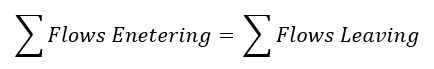Each of the available chamber types are described below.

Chamber Types

There are three main types of chambers in Flow Simulator: the plenum, the momentum balance, and the inertial chamber. There is also a vortex chamber, which is only used to store data for vortex segments and as cavity chambers. The elevation chamber (account for potential height change) is a subtype of the momentum chamber.

Plenum Chamber

The plenum chamber is used to model a region in which there is no significant velocity head present. In this case any velocity head of flow entering the chamber is dissipated and the total pressure in the chamber is equal to the static pressure. The plenum chamber is assumed to be rotating at the same speed as all of its attached elements. Therefore, all of its attached elements must be rotating at the same speed (or all elements can be stationary).

Momentum Chamber

In the momentum balance chamber, the mixed total pressure head of all flows entering the chamber is computed and resolved to give a vector flow direction. In this calculation, the direction of each incoming stream is defined by the element angles specified for the element from which the flow enters. Thereafter, according to the angular alignment of an element with flow leaving the chamber with this chamber flow direction, the appropriate component of total pressure in the direction of the element is used to drive flow from the chamber into the element. For non-rotating components, selection of the coordinate system directions relative to the engine axis is arbitrary where momentum chambers are concerned since it is only relative angle between elements that determines the inlet component of total pressure to each element. For rotating components, the coordinate system direction is not arbitrary. The same θ and ϕ angle convention as used for inertial chambers should be used so correct swirl velocities are calculated. However, it is advisable to only use momentum chambers when the swirl velocity is 0 (stationary components such as piping) or when the swirl velocity is the same as the metal rotation (XK=1, air is “onboard” the rotor). The momentum chamber is assumed to be rotating at the same speed as all of its attached elements. Therefore, all of its attached elements must be rotating at the same speed (or all elements can be stationary). Caution must be used with momentum balance chambers since the computed mixed total momentum is only valid for the total exit area of the elements having flow entering a downstream chamber. Flow Simulator assumes that the momentum of the mixing flows does not dissipate even if the downstream element(s) have a larger flow area than this. Another way of looking at this is that Flow Simulator assumes a perfect diffuser if the combined flow area of the downstream element inlets is greater than the combined exit areas of the upstream elements.

Inertial Chamber

The inertial chamber is used to model the effects of tangentially swirling air or relative tangential velocity differences between air and a rotor. The flow direction coordinates for inertial chambers are three-dimensional and follow the convention, based on the definitions in the Coordinate System section, of Z as the axial direction, U as the tangential direction and R as the radial direction. The corresponding angles are Theta (θ) in the tangential direction and Phi (ϕ) in the radial direction. The inertial chamber must be used to connect elements rotating at different speeds.

Vortex Chamber

The vortex chamber was created to facilitate the new vortex element type. No elements can use a vortex chamber as the upstream or downstream chamber. A vortex chamber’s main use is store data (XK, P, T) at vortex segments and stations. A vortex chamber stores temperature in the absolute frame like an inertial chamber. The chamber centric cavity modelling in Flow Simulator requires that a chamber be associated with each cavity. Only chambers in the absolute reference frame (inertial and vortex chambers) can be associated with a cavity.

## Chamber Inputs

The following table provides a description of Flow Simulator chamber variables. The variables are listed in the order they appear as columns of the “Chamber Data” section of a Flow Simulator model database.

CHAMBER INPUT VARIABLES
Index UI Name (.flo Label) Description
1 none A simple sequential index for the convenience of users looking at the file.
2 Chamber Number (CHNUM) Chamber identification number.
3 Type (TYP) Chamber type.

The TYP variable can be up to 3 characters. The presence of ‘-’ indicates the chamber is inactive. The presence of ‘B’ indicates the chamber is a boundary chamber. The final character indicates which type of chamber: plenum ‘P’, momentum ‘M’, inertial ‘I’, elevation ‘E’, or vortex ‘V’. Vortex chambers cannot serve as boundaries.

Examples are: P, M, E, I, BP, BM, BE, BI, V, -P, -M, -E, -I, -V, -BP, -BM, -BI.

4 Calculate Temperature (TFIX) Identifies if the chamber temperature is fixed at the TOTTEMP value or is calculated by the solver. The values are different for internal (non-boundary) and boundary chambers.
Internal:
• 0: temperature calculated by FS (default)
• 1: temperature is fixed
• >1: temperature is fixed for TFIX number of iterations*
Boundary:
• -1: temperature calculated by Flow Simulator, if the boundary chamber has flow entering
• 0 or 1: temperature is fixed (default)
• >1: temperature is fixed for TFIX number of iterations*

* If TFIX is greater than 1, then the chamber temperature is held fixed for TFIX number of iterations. If the model has not already converged, the chamber temperature is allowed to vary (in other words, calculated by Flow Simulator) thereafter.

5 Relative Total Pressure (TOTPRES) Chamber total pressure (psia)

For internal chambers, the TOTPRES input is used as an initial guess. For boundary chambers, TOTPRES is the boundary value.

6 Static Pressure (STATPRES) Chamber static pressure (psia)

For internal chambers, the STATPRES input is used as an initial guess. For boundary chambers, STATPRES is the boundary value.

7 Relative Total Temperature (TOTTEMP) Chamber fluid total temperature (deg F)

This is either an initial guess or a fixed chamber temperature, depending on the value of TFIX.

8 Mach Number (XMRES) Chamber fluid Mach number.

If the chamber is a plenum chamber, then the XMRES input will be overwritten and set to 0. For internal chambers, the XMRES input is used as an initial guess.

9 Velocity Tangential Angle (THTARES) Chamber fluid Theta flow direction angle.

If the chamber is a plenum chamber, the THTARES input will be overwritten and set to 0 since it is undefined when the fluid velocity is 0. For internal chambers, THTARES is used as an initial guess.

10 Velocity Radial Angle (PHIRES) Chamber fluid Phi flow direction angle.

If the chamber is a plenum chamber, the PHIRES input will be overwritten and set to 0 since it is undefined when the fluid velocity is 0. For internal chambers, PHIRES is used as an initial guess.

11 Material (FS) This value identifies the type of fluid in the chamber. It must be set for boundary chambers (and accumulators and tanks). The fluid material for non-boundary chambers is automatically determined in the solver so FS is ignored.
Some typical FS values are:
• FS=0: ideal air, also needs PROPS=0
• 0<FS<1: mixture of air and steam (from built-in ASME properties table), FS indicates the mass fraction of the steam, also needs PROPS=0)
• FS=1: steam (from built-in ASME properties table), also needs PROPS=0)
• FS=30:Liquid Water
• FS=31: Jet-A
• FS=32:MIL_PRF_23699 Oil
• FS=33: MIL-L-7808 Oil
• FS=35: VG-32 Oil
• FS=40.xxx: Ethylene Glycol - Water Mixture, xxx=mass fraction of EG
• FS=41.xxx: Propylene Glycol - Water Mixture, xxx=mass fraction of PG
• FS>1000: Chamber fluid can be found in a component with number FS, used with custom fluids and fluids from Coolprop.
12 For Forward Flow (CH_XK_FWD) Chamber fluid swirl factor (XK). Swirl factor is the ratio of the fluid absolute tangential velocity to the chamber’s reference tangential velocity.

For vortex chambers, this is the swirl value that is used when flow is in the forward direction through the vortex element.

For non-vortex chambers, this is the swirl value that is delivered to elements with this chamber as their upstream chamber when the element flow is in the forward direction.

CH_XK_FWD is used as an initial guess if a cavity is attached to this chamber or if the XKFIX value is 0.

CH_XK_FWD takes precedence over the vortex element XK_FWD variable.

13 For Reversed Flow (CH_XK_REV) Chamber fluid swirl factor (XK). Swirl factor is the ratio of the fluid absolute tangential velocity to the chamber’s reference tangential velocity.

For vortex chambers, this is the swirl value that is used when flow is in the reverse direction through the vortex element.

For non-vortex chambers, this is the swirl value that is delivered to elements with this chamber as their user-defined downstream chamber when the element flow is reversed.

CH_XK_REV is used as an initial guess if a cavity is attached to this chamber or if the XKFIX value is zero.

CH_XK_REV takes precedence over the vortex element XK_REV variable.

14 Chamber XK (XKFIX) Identifies if the chamber swirl (XK) is fixed at the CH_XK_FWD and CH_XK_REV values or is calculated by the solver.

The values are different for internal (non-boundary) and boundary chambers.

Internal:
• 0: swirl calculated by Flow Simulator (default)
• 1: swirl is fixed
Boundary:
• 0 or 1: swirl is fixed (default)
• 2: swirl calculated by Flow Simulator

If a cavity is attached to this chamber, Flow Simulator always calculates a swirl, regardless of user input.

15 Heat Added or Removed (CH_QIN) Specifies heat input at the chamber.

Positive values indicate heat is added. Negative values indicate heat is being withdrawn. This heat addition or extraction is independent from cavity and element related heat (BTU/sec).

16 Rotor Index (RTR_IDX) Points to the ELERPM value that is used as the reference rotation (for multiplying by CH_XK_FWD, CH_XK_REV, XK_FWD, and XK_REV).

For inertial and vortex chambers, the user choice of RTR_IDX is used.

For plenum and momentum chambers, Flow Simulator corrects RTR_IDX to agree with neighbouring elements.

The vortex RTR_IDX is also required to agree with the nearby chamber RTR_IDX values.

An error or warning will be issued in the convhis_fi.dat file when Flow Simulator detects a mismatch.

17 Height (HEIGHT) Chamber Height used for Elevation Chamber subtype (inch).
18 Fluid Quality (Quality) The fluid quality (unitless). 0=all liquid and 1=all gas.
• -999: The chamber fluid quality is not specified. (default)
• -1<=Quality
• <0: This quality is an initial guess. The solver takes the absolute value of the quality input as the initial guess.
• 0<=Quality<=1: The chamber quality is fixed to this value.

Order of Precedence for Chamber Inputs

The following table lays out the methodology that Flow Simulator uses to establish the initial chamber properties based on the chamber type and user specified chamber inputs.

ORDER ACTION
1 If you do not specify the chamber Mach number, then (MACH = 0.):

Fluid swirl (CH_XK_FWD), radius (CH_RAD), and rotational speed (RTR_IDX) are used to calculate the chamber Mach number (MACH).

(For plenum chambers, MACH = 0.)

2 If you do not specify the chamber static pressure, then (STATPRES = 0.):

Total pressure (TOTPRES) and Mach number (MACH) are used to determine the chamber static pressure (STATPRES) and resolved fluid velocity magnitude (VRES).

(For plenum chambers, STATPRES = TOTPRES, MACH = 0, and VRES = 0.)

3 If user does specify the chamber static pressure, then (STATPRES ≠ 0.):

STATPRES and Mach number (MACH) are used to determine the chamber total pressure (TOTPRES) and resolved fluid velocity magnitude (VRES).

(For plenum chambers, TOTPRES = STATPRES, MACH = 0, and VRES = 0.)

4 If a fixed quality is specified, the chamber temperature is calculated using the chamber static pressure and the quality. TOTTEMP is ignored.

## Chamber Theory Manual

The state of a Flow Simulator chamber is represented by the following variables.

PTC(i): Total pressure

PSC(i): Static pressure

TTC(i): Total temperature

XMRES(i): Resultant Mach number

FS(i): Secondary fluid mass fraction

VRES(i): Magnitude of flow velocity

THRES(i): Tangential flow angle

CPRES(i): Specific heat

GRES(i): Specific heat ratio

RRES(i): Gas constant

PTC, PSC, TTC, THRES, PHRES, XMRES and FS are the chamber variables contained in the Flow Simulator input files and printed in the Flow Simulator output files. By definition, the residual velocity is zero in a plenum chamber and the total pressure is equal to the static pressure. Non-zero flow angles specified in the input for plenum chambers are reset to zero by Flow Simulator. Beyond the possibility of non-equal total and static pressures in plenum chambers, other inconsistencies can exist in the input data for a chamber. Flow Simulator uses a hierarchy to resolve such conflicts. The static pressure, PSC, and Mach number, XMRES, are treated as the primary variables. Values of XMRES specified in the input file must not be negative and for plenum chambers they must be zero. Invalid values of XMRES will be set to zero. If PSC is not greater than zero and a value greater than zero is input for the total pressure, PTC, then total pressure will be used. Warning messages are printed to the conhist_fi.dat' file when chambers with inconsistent input data are found.

Recalculating the Chamber State

Fluid state in each chamber, with the exception of boundary chambers, is recalculated for each iteration as the solution progresses. The total and static pressure in the non-boundary chambers are the independent parameters varied by Flow Simulator to balance the flows in the circuit. The remaining chamber variables, other than those specified as fixed by some other input to the model, are dependent variables. To calculate the dependent chamber variables Flow Simulator employs the data of all the element flows entering the chamber.

For each element flow the following data is known,

W: Element flow rate

SFE: Secondary fluid mass fraction in element flow

JC: Downstream chamber number

NED: Number of element flows entering downstream chamber

TTE: Total temperature relative to the chamber rotational speed

XCP: Specific heat

PHEX: Radial flow angle relative to the chamber

THEX: Tangential flow angle relative to the chamber

VXE: Magnitude of flow velocity relative to the chamber rotational speed

(The value for XCP is obtained from the properties subroutine using TTE, SFE and the static pressure in the chamber (PSC))

The above data for each element with flow entering chamber is summed to give the following total values: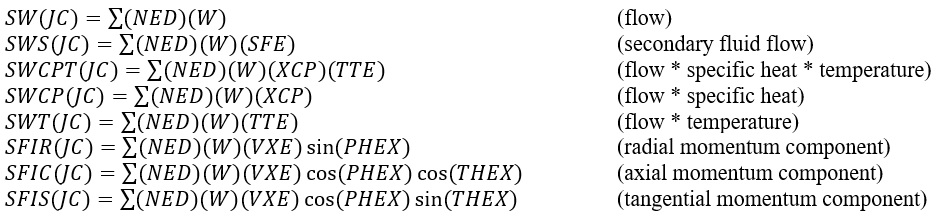The dependent chamber values are then computed from these flow-weighted values. If a chamber has no inflow, none of the dependent chamber values are updated but their values from the previous iteration are retained for use. If there is inflow into a chamber, recalculation of the properties is done.

The secondary fluid mass fraction is simply the ratio of the secondary fluid flow into the chamber to the total inflow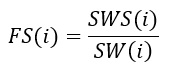The temperature of the chamber is computed as a flow and specific heat ratio weighted average. Since specific heat is itself temperature dependent, an average value between that represented by the sums calculated for the incoming flows and the specific heat of at the final mixed chamber temperature is used. This is accomplished by a simple three-loop iteration process. Initially TTOT is calculated as: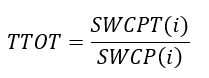Following this two more passes are made using the equation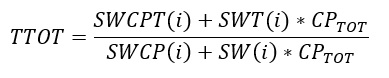CPTOT is obtained from the property’s routine using the then current value of TTOT. To complete the calculation the temperature is calculated as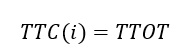Once the temperature has been established the properties routine is called to establish new values for specific heat, CPRES(i), specific heat ratio, GRES(i) and gas constant, RRES(i). The computed values of the velocity and flow direction angles depend on the type of chamber as described in the following sections.

Velocity and Flow Angles in Plenum Chambers

Since there is no velocity head associated with a plenum chamber, the values of Mach number, resultant velocity, and flow angles are not updated but remain set at zero. Hence for each plenum chamber (i) we have,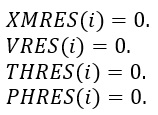Velocity and Flow Angles in Momentum and Inertial Chambers

The momentum and inertial chambers, while used differently during element flow calculations, are handled similarly when the chamber velocity and flow angles are calculated. The tangential flow angle is computed from the axial and tangential components of mixed total momentum,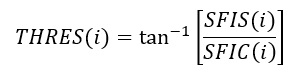The radial flow angle, which is measured in the radial direction from the axial-tangential component of velocity, is computed from all three components of mixed total momentum,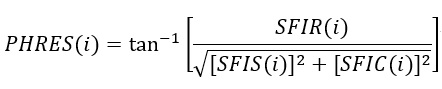Likewise, the velocity is computed from the three components of the mixed total momentum and the total flow rate,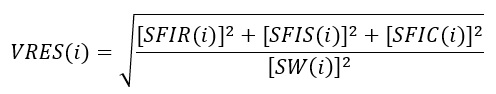The resultant Mach number is computed from Mach number routine using TTC, GRES, RRES and VRES.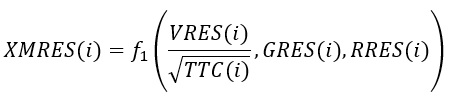For inertial chambers, XMRES is not limited in magnitude and may be greater than 1.0 if VRES is sufficiently large. For momentum chambers, the GENDAT input variable ISUPER determines whether XMRES is permitted to be supersonic. The default value of ISUPER = 0 limits the maximum value of XMRES for momentum chambers to 1.0. The chamber XK (swirl ratio) is not a primary chamber variable but it is calculated in the same routine as the rest of the chamber data so will be described here. The calculated XK’s are calculated and reported with respect to the absolute reference frame for all chamber types. The XK calculation does depend on the chamber type, however. The XK of a plenum chamber should always be 0 or 1 since a plenum chamber is rotating with its attached elements and the through flow velocity from the attached elements is dissipated. The XK for a momentum chamber is based on the velocity in the chamber, VRES, the flow angles, θ and ϕ and the chamber rotation. The XK for an inertial chamber is based on the velocity in the chamber, VRES, the flow angles θ and ϕ. If the inertial chamber is associated with a cavity, the calculated cavity XK is used to set the chambers tangential velocity by recalculating SFIS.

The chamber radius, CH_RAD, is determined by the average radius of certain elements attached to the chamber. The elements used for averaging are the following: rotating tube, rotating orifice (Conventional, Effective Area Orifice, and CDComp), Vermes seal, and a vortex element. All element attached to a chamber should have the same radius, but if they do not a warning will be written. If the chamber is a vortex type chamber it will not have any attached elements. A Vortex chamber will get its radius from the average of the cavity surface radial extent (for vortex chambers with cavities) or from the vortex element stations.

Chamber XK

The chamber swirl ratio, CH_XK_FWD (for forward flow) and CH_XK_REV (for reverse flow), is also calculated for use in the cavity and vortex modules and output to the *.res file. The swirl is relative to the user provided ELERPM(CHM_RTR_IDX). The chamber XK is always an absolute value (V_θ used in XK calculation is absolute).

## Chamber Outputs

The following table provides a description of Flow Simulator chamber output variables. The variables are listed in the order they appear as columns *.res file.

Name Description Units
CHAM Chamber identification number. (ID number)
(CH)TYP Chamber type.

P: Plenum

M: Momentum

I: Inertial

V: Vortex

(flag)
ERROR1 Flow imbalance at the chamber as a percentage of the total flow through the chamber. (%)
PTC Chamber total pressure.

Relative for plenum and momentum chambers.

Absolute for inertial and vortex chambers.

psi, mPa
PSC Chamber static pressure. psi, mPa
TTC Chamber total temperature.

Relative for plenum and momentum chambers.

Absolute for inertial and vortex chambers.

deg F, K
THRES Resolved theta angle of flows entering the chamber.

Relative for plenum and momentum chambers.

Absolute for inertial and vortex chambers.

deg
PHIRES Resolved phi angle of flows entering the chamber.

Relative for plenum and momentum chambers.

Absolute for inertial and vortex chambers.

deg
MACH Resolved Mach number of flows entering the chamber.

Relative for plenum and momentum chambers.

Absolute for inertial and vortex chambers.

(unitless)
FS Secondary fluid mass fraction. (unitless)
XK Fluid rotation as a fraction of the chamber’s reference rotation. (unitless)
RTR A pointer to the chamber’s reference rotation (RPM) for fluid rotation calculations.

0: Stationary.

1: ELERPM(1).

2: ELERPM(2).

3: ELERPM (3).

(index)
T_FRM A pointer to the chamber’s reference rotation (RPM) for fluid temperature calculations.

0: Stationary.

1: ELERPM(1).

2: ELERPM(2).

3: ELERPM (3).

-1: Rotating with the fluid from the upstream element.

-2: Rotating with the fluid from the downstream element.

(index)
CH_QIN Heat input to the chamber.

Positive values indicate heat added to the fluid; negative values indicate heat removed.

BTU/s, W
TS Chamber static temperature. deg F, K
TT_ABS Chamber absolute total temperature. deg F, K
TT_REL Chamber total temperature relative to the rotational reference frame (i.e. rotor). deg F, K
TFIX Flag that tells how chamber temperature was fixed:

0: Variable temperature (calculated by Flow Simulator).

1: Permanently fixed chamber temperature.

> 1: Chamber temperature is held fixed for TFIX number of iterations and, if the model has not already converged, the chamber temperature is allowed to vary (i.e. be calculated by Flow Simulator) thereafter.

(flag)
VRES Resolved fluid velocity magnitude of flows entering the chamber.

Relative for plenum and momentum chambers.

Absolute for inertial and vortex chambers.

ft/s, m/s
VAX Component of chamber resolved velocity in the axial direction. ft/s, m/s
VRAD Component of chamber resolved velocity in the radial direction. ft/s, m/s
VABS_TAN Absolute fluid tangential velocity.

(For an inertial or vortex chamber, this will be the same as VRES_TAN.)

ft/s, m/s
VREL_TAN Fluid tangential velocity relative to the rotational reference frame (i.e. rotor).

(For a plenum or momentum chamber, this will be the same as VRES_TAN.)

ft/s, m/s
VRES_TAN Component of chamber resolved velocity in the tangential direction. ft/s, m/s
CH_RPM Reference rotational speed of the chamber (based on T_FRM). rev/min
Height Chamber Height inch, mm
TOT_ENTH Total Enthalpy. This column is written if enthalpy is used for the energy equations.
FLUID The source of the fluid properties for this chamber.
Note: Details of the fluid properties are in the *.props file.